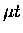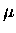Next: fusion Up: Systematic effects Previous: Cut efficiency correction

### Nitrogen contamination

If there was a contamination of heavier elements in the emission layer, the yield ofwould be reduced due to muon transfer from the proton to the heavy element. In some of the runs, we observed muonic X rays from muonic nitrogen atomic transitions, indicating contamination of the target. From the analysis of the Lyman series X rays, the contamination level was estimated to be a few ppm level by Francoise Mulhauser [83,231]. In the constant rate, infinite medium approximation, the probability ofproduction can be expressed as:

 (107)

where cN2 is the concentration of N2 molecules, and the muon transfer rate from a proton to a nitrogen atom. Using the proton to triton transfer rates-1, andformation rates-1, both obtained from our earlier measurements in solids , together with the transfer rate to nitrogen s from Ref.  (measured in a gas), we can estimate the reduction factor for theproduction , which is normalized to the pure target yield . Table 8.12 presents the correction factor for two series of target sets which are of relevance in our analysis.

Table 8.9: Theproduction probability with possible nitrogen contamination, and the reduction factor , normalized to a pure emission target .
 Target ID cN2 (Ref. ) SETct=0.1% (a) II-1 to II-4 3.3+6.8-3.3 ppm 0.621+0.14-0.28 SETct=0.1% (b) II-6 to II-11 2.3+4.6-2.3 ppm 0.611+0.11-0.23

We note that the estimate of nitrogen contamination by Mulhauser gives asymmetric errors as quoted in the table, but we take the average of two extreme values of 1error bars for convenience in the data treatment. In our analysis, we assume that reduction inemission is proportional to the factor given here, neglecting the effects of the transfer, which is presumably much smaller.Next: fusion Up: Systematic effects Previous: Cut efficiency correction